Fluid flow homework Help at TutorEye

## Fluid flow:

Fluid flow is a discipline of fluid dynamics which deals in the motion of fluid and its behaviour to external forces. It involves flow of the fluid subjected to unbalanced forces. Flow of fluid is further classified according to the nature, rotational and other time dependent property. The unbalanced forces affect the flow in its direction, speed and streamline.

## Fluid flow Sample Questions:

Question 1: __________ method is used to analyse the fluid flow in fluid mechanics.

a) Lagrangian               b) Eulerian
c) Both (a) and (b)        d) None of these

Explanation: In a fluid flow, the characteristic study is about the general state of motion at various points, which is an eulerian approach.

Question 2: The fluid flow is __________ inside the boundary layer and __________ outside.

a) rotational, irrotational           b) irrotational, rotational
c) rotational, rotational             d) irrotational, irrotational

Explanation: Inside the boundary layer, viscosity is predominant, which results in the development of shear stress. The rotation is associated with the shear stress. In the application of torque fluid particles undergo rotation.Viscosity is negligible outside the boundary layer, which doesn't result in the development of shear stress and torque. Therefore, flow is irrotational outside the boundary layer.

Question 3: In a steady flow, which of the following lines are identical?

a) Streamline                 b) Streakline
c) Pathline                     d) All of the above

Explanation: In a steady flow, velocity at any point of time remains constant. Therefore, all the lines streamline, streakline and pathline are identical without any geometrical difference.

Question 4: Find the condition for the flow field to be continuous in the given two dimensional flow?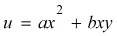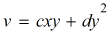a)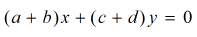b)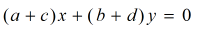c)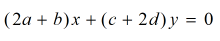d)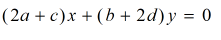Answer:Explanation: The condition for the flow to be continuous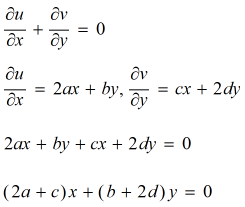Question 5: The continuity equation of fluid flow is based on the principle of ____________

a) conservation of momentum     b) conservation of energy
c) conservation of mass               d) all of the above

Explanation: If there is no addition or removal of mass from any cross-section in the fluid flow, then the flow of mass remains constant. This states the principle of conservation of mass.

Question 6: In the continuity equation, fluid flow is assumed to be _________

Explanation: Flow is assumed to be steady in most of the cases of fluid flow. The rate of flow is constant w.r.t time.

Question 7: Which of the following fluid flow is along a curvilinear path?

a) Circular flow             b) Sink flow
c) Rotational flow         d) Vortex flow

Explanation: Vortex flow is the flow of fluid along a curvilinear or curved path.

Question 8: In an incompressible flow, what is the effect of reduction in area on velocity?

a) Increases                b) Decreases
c) Both (a) and (b)     d) None of these

Explanation: In the continuity equation, area is inversely proportional to the velocity. Therefore, a decrease in the area results in increase of velocity.

Question 9: _____________ is defined as the rate of change of velocity due to change of position of fluid particles.

a) Acceleration                 b) Convective acceleration
c) Local acceleration        d) None of these

Explanation: The rate of change of velocity with respect to change in the position of fluid particles is known as convective acceleration.

Question 10: The nature of streamline in free vortex flow is ___________.

a) Linear                      b) Non-Linear
c) Non-concentric        d) Concentric

Explanation: Streamline in free vortex flow is circular with a common center. Hence, nature of streamline is concentric.